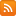CONTÁCTENOS - 91 575 78 24Estás en www.librosingenieria.com
Si no encuentra un libro lo buscamos por Ud.
91 575 78 24

## CESTA DE LA COMPRA

Tiene 0 productos en su cesta Importe total: 0
Wavelet Analysis in Civil Engineering

# Wavelet Analysis in Civil Engineering

## Descripción

The principles of wavelets can be applied to a range of problems in civil engineering structures, such as earthquake-induced vibration analysis, bridge vibrations, and damage identification. This book is particularly useful for graduate students and researchers in vibration analysis, especially those dealing with random vibrations.

## Características

• ISBN: 978-1482210552
• Páginas: 224
• Tamaño: 17x24
• Edición:
• Idioma: Inglés
• Año: 2015

# Contenido Wavelet Analysis in Civil Engineering

Wavelets as a Powerful Signal Processing Tool

The principles of wavelets can be applied to a range of problems in civil engineering structures, such as earthquake-induced vibration analysis, bridge vibrations, and damage identification. This book is particularly useful for graduate students and researchers in vibration analysis, especially those dealing with random vibrations.

Wavelet Analysis in Civil Engineering explains the importance of wavelets in analyzing nonstationarities in ground motions. The example of a tank is considered to develop the problem and the model (based on linear assumptions) and several case studies are explored—fixed base, flexible base, lateral and rocking motions of foundations, with and without fluid—to explain how to account for ground motion nonstationarities. Bridge vibrations caused by vehicle passage are explored, as is structural damage identification. Wavelet analytic techniques starting from single degree of freedom systems to multiple degree of freedom systems are set out and detailed solutions of more complicated problems involving soil and fluid interactions are presented. Separate chapters have been devoted to explaining the basic principles of the wavelet-based random nonstationary vibration analysis of nonlinear systems, including probabilistic analysis.

Comprised of seven chapters, this text:

Introduces the concept and utility of wavelet transform
Describes the discretization of ground motions using wavelet coefficients
Explains how to characterize nonstationary ground motions using statistical functionals of wavelet coefficients of seismic accelerations
Develops the formulation of a linear single-degree-of-freedom system
Shows stepwise development of the formulation of a structure idealized as a linear multi-degree-of-freedom system in terms of wavelet coefficients
Defines wavelet domain formulation of a nonlinear single-degree-of-freedom system
Introduces the concept of probability in wavelet-based theoretical formulation of a nonlinear two-degree-of-freedom system
Covers a variety of case studies highlighting diverse applications

Wavelet Analysis in Civil Engineering explains the importance of wavelets in terms of non-stationarities of ground motions, explores the application of wavelet analytic techniques, and is an excellentresource for users addressing wavelets for the first time.

Contents

Preface

Introduction

1 Introduction to wavelets

1.1 History of wavelets
1.2 Fourier transform
1.3 Random vibration
1.4 Wavelet analysis
1.5 A brief review of wavelet properties
1.5.1 Continuous and discrete wavelet transform
1.5.2 Vanishing moments
1.5.3 Uncertainty principle

2 Vibration analysis of SDOF and MDOF systems in the wavelet domain

2.1 Wavelet-based discretization of ground motions
2.2 Time–frequency characteristics of wavelets
2.3 Formulation of SDOF system equation in wavelet domain
2.4 Wavelet basis function for ground motion process
2.5 Wavelet domain stochastic response of SDOF system
2.6 Statistical parameters and nonstationary peak responses
2.7 Wavelet domain stochastic response of MDOF system

3 Ground motion characterization and PSA response of SDOF system

3.1 Characterization of ground motions
3.2 PSA response spectrum
3.3 Time history simulation by the Runge–Kutta fourth-order method
3.4 Fixed-base analysis of a tank
3.4.1 Basic assumptions
3.4.2 Equations of motion
3.4.3 Wavelet domain formulation
3.5 Numerical study
3.5.1 Ground motion characterization
3.5.2 Validation – PSA response
3.5.3 Validation – structural response
3.5.4 Wavelet analysis – structural response

4 Wavelet-based analysis of linear MDOF system

4.1Description of the model
4.2 Equations of motion
4.3 Wavelet domain formulation of tank–liquid–foundation system
4.4 Wavelet-based nonstationary system responses
4.5 Solution of transfer functions
4.6 Expected largest peak response
4.7 Numerical example
4.7.1 Impulsive response
4.7.2 MDOF analysis results

5 Wavelet-based nonstationary vibration analysis of a simple nonlinear system

5.1 Nonlinear system
5.2 Duffing oscillator
5.3 Perturbation method
5.4 Solution of the Duffing equation
5.5 Nonlinear system subjected to random vibration

6 Wavelet-based probabilistic analysis

6.1 Model and soil nonlinearity
6.2 General equations of motion
6.3 Equations based on yield conditions
6.4Transfer functions
6.5 Response of the structure
6.6 Probability evaluation
6.7 Validation and results

7 General applications

7.1 B-WIM NOR signal analysis
7.1.1 Bridge and vehicle model
7.1.2 Wavelet analysis of experimental NOR data
7.2.1 Description of the analytical model
7.2.2 Numerical approach to wavelet-based damage detection
7.2.3 Finite element model
7.2.4 Wavelet-based analysis of numerical results
7.3 Soil–structure–soil interaction analysis
7.3.1 Responses at tank base
7.3.2 Finite element model of the system

Appendix: MATLAB® programmes

References
Index

## ACERCA DE LA EMPRESA